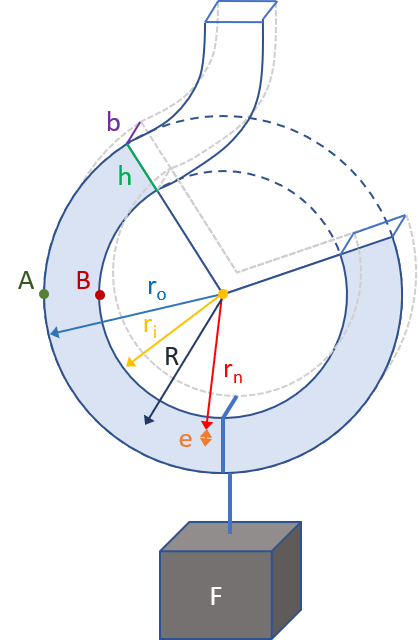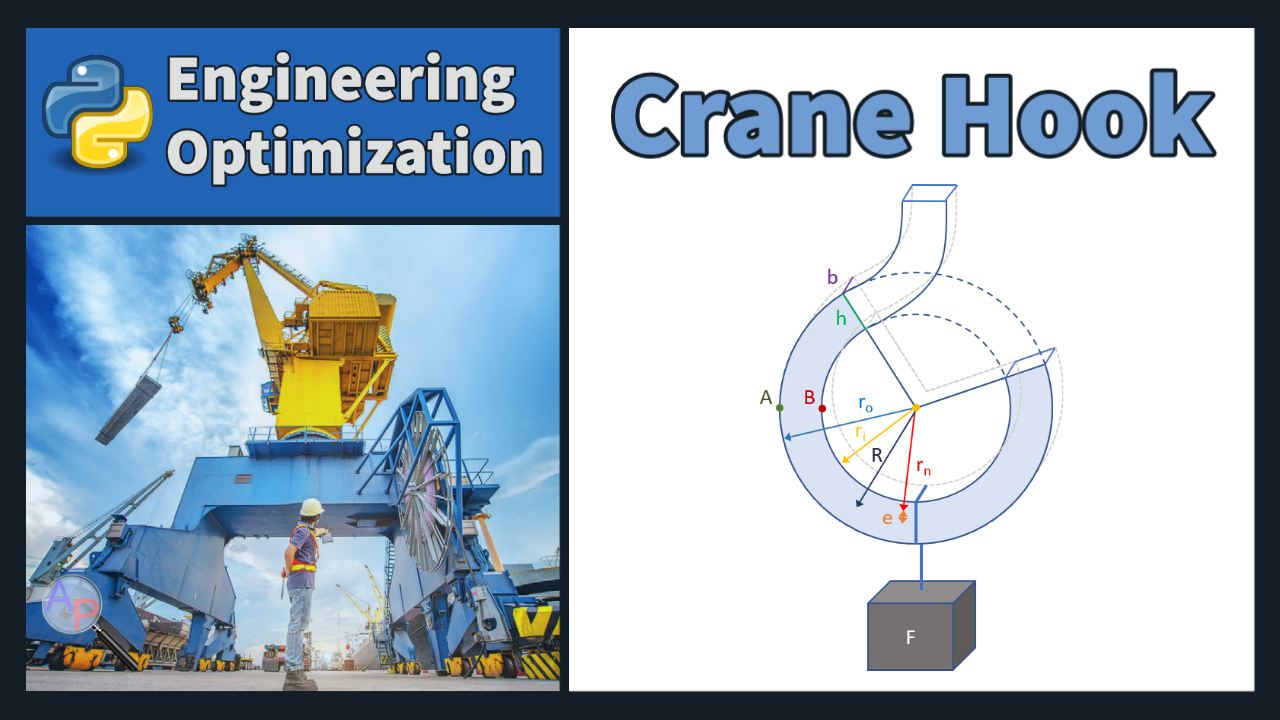## Crane Hook Design Optimization

A crane hook is used for lifting and moving heavy objects and is often found in industrial applications. Design a crane hook to carry a load F. The hook has a rectangular cross section with width b (minimum 0.2 mm) and height h.Optimize the crane hook design to minimize the volume of the hook. The hook is manufactured from a complete rectangular wire ring that is clipped and bent to give the final hook shape. The outer radius of the hook is ro and the inner radius is ri with a minimum inner diameter of 3.0 mm. The height is the difference between the outer and inner radius h=ro-ri. The bending moment is M=FR with a force F of 100 N (10.2 kg for a static load on earth). The centroid radius is R and the neutral axis radius is rn.

$$r_n = \frac{h}{\ln\left(r_o/r_i\right)}$$

The difference between the centroid radius and the neutral axis radius is e. The stress at point A is

$$\sigma_A = \frac{M \left(r_o-r_n\right)}{b\;h\;e\;r_o}$$

The stress at point B is

$$\sigma_B = \frac{M \left(r_n-r_i\right)}{b\;h\;e\;r_i}$$

The stress at points A and B should not exceed the yield strength of the steel at 430 N/mm2.

Solution

# Crane Hook optimization
from gekko import GEKKO
from numpy import pi

m = GEKKO(remote=False)

# Constants
F = 100                 # load (N)
S_y = 430               # yield strength of steel (N/mm^2)
#  Grade D fine-carbon steel (ASTM A255)

# Variables
r_o  = m.Var()          # outer radius (mm)
r_i  = m.Var(lb=1.5)    # inner radius (mm)
b    = m.Var(lb=0.2)    # hook width (mm)
V    = m.Var()          # hook volume (mm^3)

# Intermediates
h    = r_o - r_i        # hook height (mm)
R    = (r_o + r_i)/2    # radius of the centroid (mm)
r_n  = h/m.log(r_o/r_i) # radius of the neutral axis (mm)
e    = R - r_n          # R - r_n (mm)
M    = F * R            # bending moment due to the load
c_o  = r_o - r_n        # distance from outer to neutral(mm)
c_i  = r_n - r_i        # distance from inner to neutral(mm)
Area = b*h              # cross-sectional area (mm^2)
o_A = (M*c_o/(Area*e*r_o)) # outer stress
o_B = (M*c_i/(Area*e*r_i)) # inner stress

# Equations
m.Equations([
V == pi*(r_o**2-r_i**2)*b, # volume calculation
o_A < S_y,      # yield stress @ A < yield strength
o_B < S_y,      # yield stress @ B < yield strength
r_i < r_o       # constraint for feasibility
])

# Objective
m.Minimize(V)

# Solve
m.options.SOLVER = 3
m.solve()

print('Optimal Volume: ' + str(V))
print('Optimal outer radius: ' + str(r_o))
print('Optimal inner radius: ' + str(r_i))
print('Optimal hook width: ' + str(b))

The optimal solution is:

  Optimal Volume: 37.50 mm^2